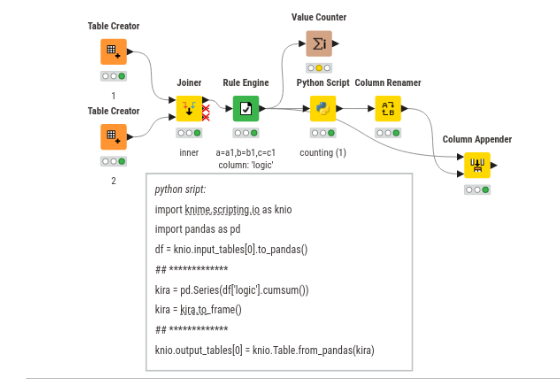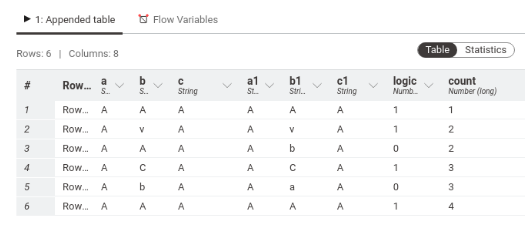# how to compare the columns and count their repeatabilty.

Hiii Everyone ,

Its my first initial days with Knime,. I hope, I will learn it a lot with your support and guidance.
My problem is following:- I have 2 tables and each table has 3 columns , such as In Table 1, there are a, b,c are columns. Whereas, In table 2 columns are a1, b1,c1. So now , if a=a1,b=b1,c=c1, then return(counting) in a seprate column (1+ increasing(1)=means 2), hint:- as we get while using loops automatic increasing 1 for every itration.
column
a b c
A A A
A v A
A A A
A C A
A b A
A A A
B c B
B f B
B g B
B B B
B h B
B k B
C C C
C l C
C e C

a1 b1 c1
A A A
A v A
A A A
A C A
A b A
A A A
B c B
B f B
B g B
B B B
B h B
B k B
C C C
C l C
C e C

I hope , Soon I will get help from you.
Thank you.

hi @NishantSingh , welcome to the community.

based on your description, i assume that you require all (a=a1,b=b1,c=c1) has to be true.
i’m using a script to imitate the counting.have a great time knimining
sorry if did not meet your expectations.
rgds

1 Like

Thank you so much for your response. Actually , I am looking forward , when a=a1and b=b1 and c=c1 then must be printed 1+ increasing 1 means totally =2 and if any of them not equal to each other such (a not equal a1,but b=b1,c=c1) should print only 1.
Little more explanation, How this workflow has to be used in work:
For future , when we have one output workflow with result of counting like
2
2
1
2
1
1
1
2

As we have to compare the table again with each other: first table we have from our last resulting table and second table we have a new table.Since we are comparing (old)a=a1(new)and (old)b=b1(new) and (old)c=c1(new)
How the result should be in the counting table
2(from last result table)+(if a=a1and b=b1 and c=c1 then 1 else no change)